Next: Resonant Scattering Up: Scattering Theory Previous: Hard-Sphere Scattering

# Low-Energy Scattering

In general, at low energies (i.e., when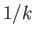is much larger than the range of the potential), partial waves with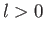make a negligible contribution to the scattering cross-section. It follows that, with a finite-range potential, only S-wave (i.e., spherically symmetric) scattering is important at such energies.

As a specific example, let us consider scattering by a finite potential well, characterized by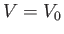for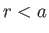, and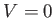for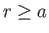. Here,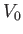is a constant. The potential is repulsive for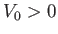, and attractive for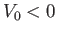. The external wavefunction is given by [see Equation (10.95)]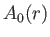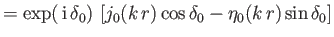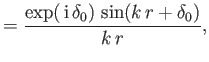(10.118)

where use has been made of Equations (10.60) and (10.61). The internal wavefunction follows from Equation (10.100). We obtain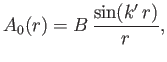(10.119)

where use has been made of the boundary condition (10.101). Here,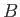is a constant, and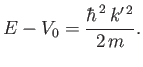(10.120)

Note that Equation (10.120) only applies when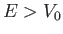. For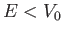, we have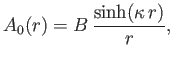(10.121)

where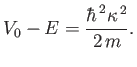(10.122)

Matching, and its radial derivative, at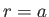yields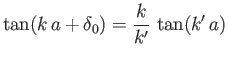(10.123)

for, and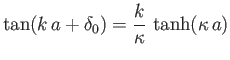(10.124)

for.

Consider an attractive potential, for which. Suppose that(i.e., the depth of the potential well is much larger than the energy of the incident particles), so that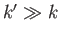. As can be seen from Equation (10.124), unless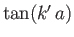becomes extremely large, the right-hand side of the equation is much less than unity, so replacing the tangent of a small quantity with the quantity itself, we obtain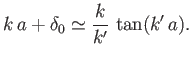(10.125)

This yields(10.126)

According to Equation (10.115), the total scattering cross-section is given by(10.127)

Now,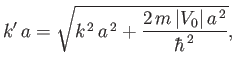(10.128)

so for sufficiently small values of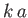,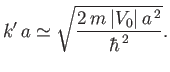(10.129)

It follows that the total (S-wave) scattering cross-section is independent of the energy of the incident particles (provided that this energy is sufficiently small).

Note that there are values of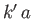(e.g.,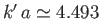) at which the scattering cross-section (10.128) vanishes, despite the very strong attraction of the potential. In reality, the cross-section is not exactly zero, because of contributions frompartial waves. But, at low incident energies, these contributions are small. It follows that there are certain values of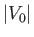,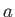, and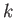that give rise to almost perfect transmission of the incident wave. This is called the Ramsauer-Townsend effect, and has been observed experimentally [88,4].Next: Resonant Scattering Up: Scattering Theory Previous: Hard-Sphere Scattering
Richard Fitzpatrick 2016-01-22